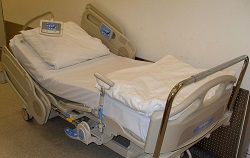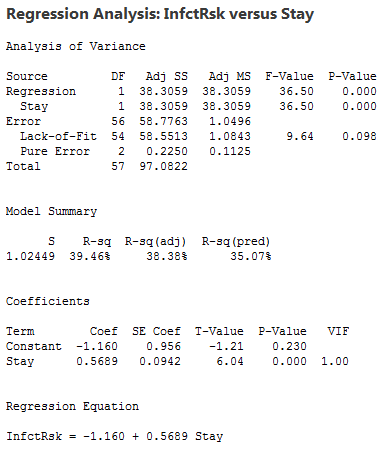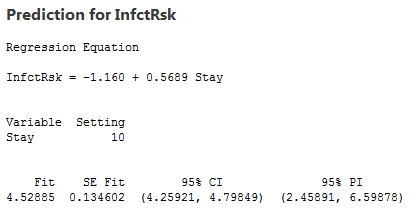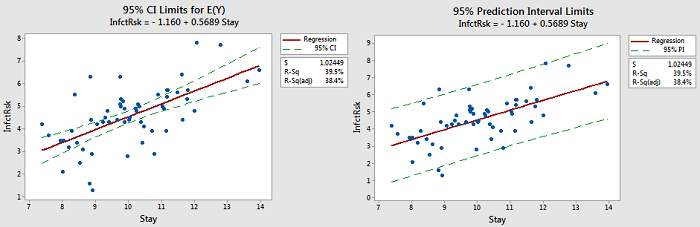# 4.12 - Further Example of Confidence and Prediction Intervals

### Hospital Infection DataThe hospital infection risk dataset consists of a sample of 113 hospitals in four regions of the U.S. The response variable is y = infection risk (percent of patients who get an infection) and the predictor variable is x = average length of stay (in days). Here we analyze n = 58 hospitals in the east and north central U.S (regions 1 and 2). [Two hospitals with extreme values for Stay have also been removed.] Statistical software output for a simple linear regression model fit to these data follows:Software output with information for x = 10.We can make the following observations:

1. For the interval given under 95% CI, we say with 95% confidence we can estimate that in hospitals in which the average length of stay is 10 days, the mean infection risk is between 4.25921 and 4.79849.
2. For the interval given under 95% PI, we say with 95% confidence that for any future hospital where the average length of stay is 10 days, the infection risk is between 2.45891 and 6.59878.
3. The value under Fit is calculated as $\hat{y} = −1.160 + 0.5689(10) = 4.529$.
4. The value under SE Fit is the standard error of $\hat{y}$ and it measures the accuracy of $\hat{y}$ as an estimate of E(Y ).
5. Since df = n − 2 = 58 − 2 = 56, the multiplier for 95% confidence is 2.00324. The 95% CI for E(Y) is calculated as $4.52885 \pm (2.00324 × 0.134602) = 4.52885 \pm 0.26964 = (4.259, 4.798)$.
6. Since S = $\sqrt{MSE}$ = 1.02449, the 95% PI is calculated as $4.52885 \pm (2.00324 × \sqrt{1.02449^2 + 0.134602^2}) = 4.52885 \pm 0.20699 = (2.459, 6.599)$.

The following figure provides plots showing the difference between a 95% CI for E(Y ) and 95% PI for y.There are also some things to note:

1. Notice that the limits for E(Y) are close to the line. The purpose for those limits is to estimate the "true" location of the line.
2. Notice that the prediction limits (on the right) bracket most of the data. Those limits describe the location of individual y-values.
3. Notice that the prediction intervals are wider than the confidence intervals. This is something that can be noted by the formulas.